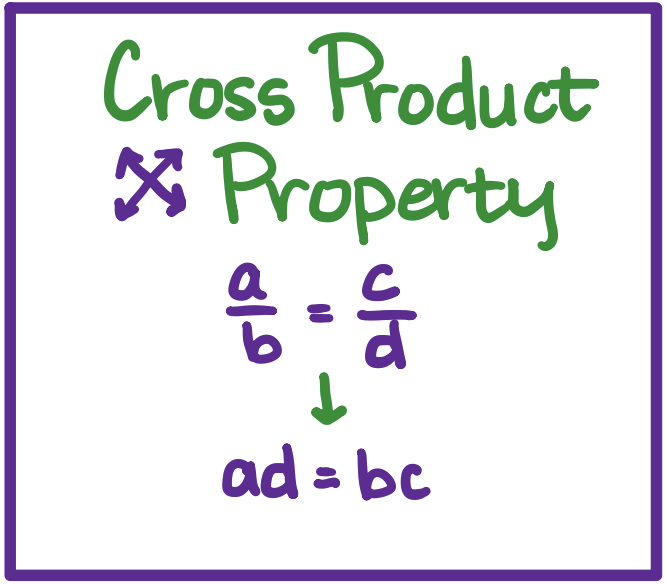# MS Math – Oct 11-15, 20216th Grade Math – Mrs. Evans

This week we will be extending our understanding and skills with fraction operations, beginning with writing decimals as fractions and fractions with decimals.  We will compare and order decimals and fractions and then we will begin to multiply fractions, learning a wonderful trick to simplify them as we multiply.  Here is a little picture to help students, and families, convert between decimals and fractions:Converting from a decimal to a fraction is done by remembering that a decimal is a portion of a factor of ten, as shown here:We understand that we can write a decimal as a fraction and a fraction as a decimal simply by remembering these simple lessons.  Here is a video that can help students who want a little more help converting from a decimal to a fraction and a fraction to a decimal:

Math Grade Math – Mrs. Evans and Mrs. VonFeldt

This week we will be working in Module 3, in which we will apply all that we learned in Modules 1 and 2 to rational numbers.  Rational numbers are any number that can be represented by a ratio of two integers (the second of which is not zero).  The most important thing to know about rational numbers is that they include decimals and fractions.  Here is a picture showing what rational numbers are:We apply the rules and logic we learned about operations with negatives to decimals and fractions.  This means we will be able to do all the basic operations to all the types of numbers!    For any students who want to review how to convert between decimals and fractions, please see the video links above (6th grade math update).

8th Grade Math – Mrs. Ernest

This week 8th graders are reviewing algebra concepts from last week and applying these ideas to graphing problems. We will be working through Chapter 2 this week, focusing on how to graph, how to graph linear equations from an x,y table and writing linear equations from word problems.

Graphing Review:

Algebra – Mrs. VonFeldt

This week, students will continue to solve and master solving one-variable equations. They will learn how to solve proportional relationships using cross products:We will also learn how to rewrite equations and formulas to be solved for a specific variable. This is often helpful to rewrite formulas with multiple variable inputs. At the end of the week, we will wrap up Chapter 2 with a team test on Thursday. The individual test will be next Tuesday.

© 2023 Assumption Catholic School | 2116 Cornwall Ave, Bellingham, WA 98225
Phone: 360.733.6133 | Fax: 360.647.4372
Email: theoffice@school.assumption.org
Dashboard | Web design and development by Olywebdev.com